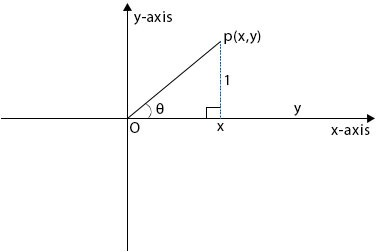### Trigonometry (III)

Mathematics SSS 1 Third Term

WEEK 7

Trigonometry (III)

Performance Objectives

Students should be able to;

1. Participate in the construction of a table of value
2. Plot the points from the table of value obtained
3. Draw the graph of trigonometry

Trigonometry ratios related to the Unit Circle

Content

1. Angles between 00 and 3600.

Consider a circle of radius one unit and centre at O, the origin, in the XY plane.The circle is called a unit circle. Point P has co-ordinate (x,y) and lOPl = 1 unit.

Line Op makes an angle of θ with Ox.

.... View More